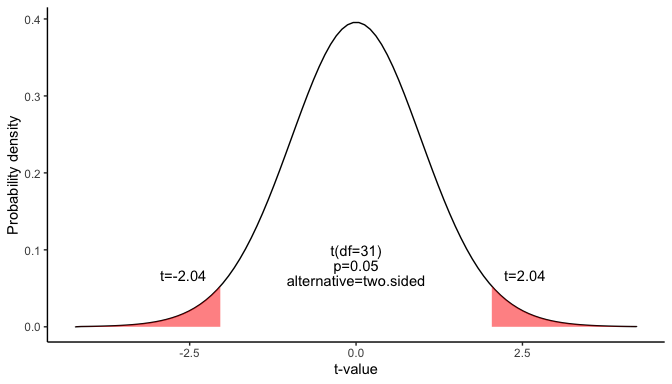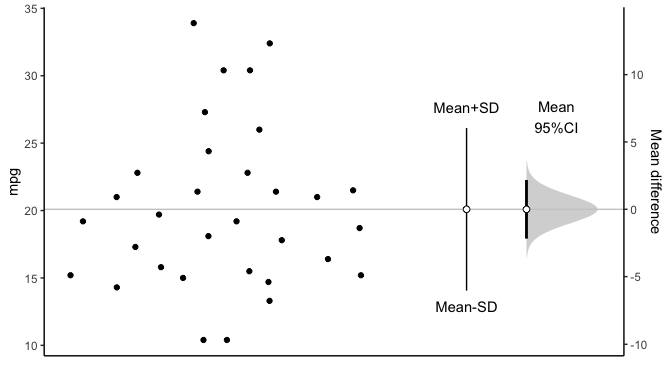# Confidence interval for a mean

This document is prepared automatically using the following R command.

 library(interpretCI)x=meanCI.data.frame(x = mtcars, mpg)interpret(x)

## Problem

 An inventor has developed a new, energy-efficient lawn mower engine. From his stock of 3200 engines, the inventor selects a simple random sample of 32 engines for testing. The engines run for an average of 20.09 minutes on a single gallon of regular gasoline, with a standard deviation of 6.03 minutes. What is the 95% confidence interval for the average minutes? (Assume that run times for the population of engines are normally distributed.

## Confidence interval of mean

The approach that we used to solve this problem is valid when the following conditions are met.

• The sampling method must be simple random sampling.

• The sampling distribution should be approximately normally distributed.

Since the above requirements are satisfied, we can use the following four-step approach to construct a confidence interval of mean.

### Raw data

The first 10 rows of the provided data is as follows.

# A tibble: 10 × 1
mpg
<dbl>
1  21
2  21
3  22.8
4  21.4
5  18.7
6  18.1
7  14.3
8  24.4
9  22.8
10  19.2

### Sample statistics

The sample size is 32, the sample mean is 20.09 and the standard error of sample is 6.03. The confidence level is 95 %.

### Find the margin of error

Since we do not know the standard deviation of the population, we cannot compute the standard deviation of the sample mean; instead, we compute the standard error (SE). Because the sample size is much smaller than the population size, we can use the “approximate” formula for the standard error.

$SE= \frac{s}{\sqrt{n}}$ where s is the standard deviation of the sample, n is the sample size.

$SE=\frac{6.03}{\sqrt{32}}=1.07$ Find the critical probability(p*):

$p*=1-\alpha/2=1-0.05/2=0.975$

The degree of freedom(df) is: $df=n-1=32-1=31$

The critical value is the t statistic having 31 degrees of freedom and a cumulative probability equal to 0.975. From the t Distribution table, we find that the critical value is 2.04.

 df 0.4 0.25 0.1 0.05 0.025 0.01 0.005 0.001 29 -0.256 -0.683 -1.311 -1.699 -2.045 -2.462 -2.756 -3.396 30 -0.256 -0.683 -1.31 -1.697 -2.042 -2.457 -2.75 -3.385 31 -0.256 -0.682 -1.309 -1.696 -2.04 -2.453 -2.744 -3.375 32 -0.255 -0.682 -1.309 -1.694 -2.037 -2.449 -2.738 -3.365

$qt(p,df)=qt(0.975,31)=2.04$

The graph shows the $$\alpha$$ values are the tail areas of the distribution.Compute margin of error(ME):

$ME=critical\ value \times SE$ $ME=2.04 \times 1.065=2.173$

Specify the confidence interval. The range of the confidence interval is defined by the sample statistic $$\pm$$margin of error. And the uncertainty is denoted by the confidence level.

### Confidence interval of the mean

Therefore, the 95% confidence interval is 17.92 to 22.26. That is, we are 95% confident that the true population mean is in the range 17.92 to 22.26.

### Plot

You can visualize the mean difference:### Result of meanCI()


call: meanCI.data.frame(x = mtcars, mpg)
method: One sample t-test
alternative hypothesis:
true mean  is not equal to  0

Results
# A tibble: 1 × 7
m        se     DF    lower    upper    t      p
<chr>    <chr>  <chr> <chr>    <chr>    <chr>  <chr>
1 20.09062 1.0654 31    17.91768 22.26357 18.857 < 2.2e-16

### Reference

The contents of this document are modified from StatTrek.com. Berman H.B., “AP Statistics Tutorial”, [online] Available at: https://stattrek.com/estimation/confidence-interval-mean.aspx?tutorial=AP URL[Accessed Data: 1/23/2022].